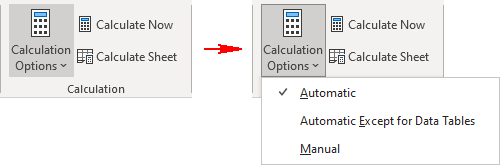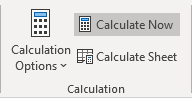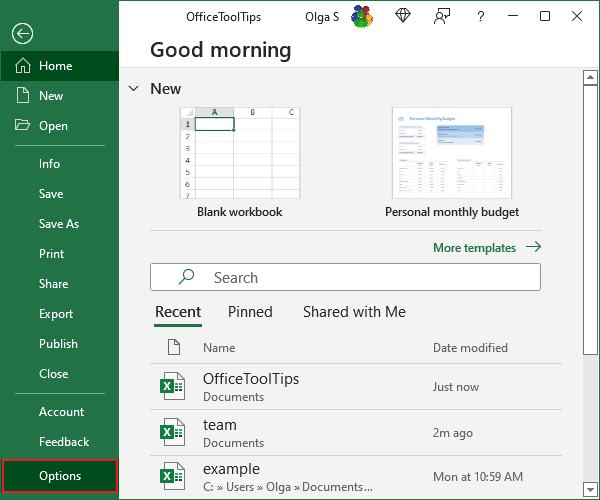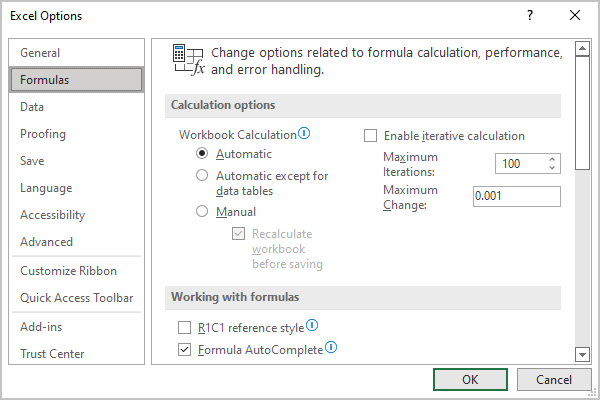# Recalculating the worksheet

Excel
By default, Excel automatic recalculation feature is enabled. Thus, all formulas in the workbook sheets recalculate immediately after data dependencies change. Automatic recalculation of all formulas also occurs when opening a workbook.

## Calculation options in Excel

Excel automatically recalculates only those formulas that depend on the changed data (except for opening the workbook) to avoid wasting time and not reduce work speed.

However, Excel provides an option to change this behavior to stop Excel calculations.

To change Excel calculation options, on the Formulas tab, in the Calculation group, click the Calculation Options button, then select any of the options:• The Automatic option, used by default, means that Excel will automatically recalculate any dependent formulas every time worksheet data changes.
• The Automatic Except for Data Tables option means that Excel will automatically recalculate any dependent formulas except data tables.

Note: This option will disable Excel calculations for data tables only, while a regular Excel spreadsheet will perform automatic calculations in Excel.

• The Manual option will disable calculations in Excel. The open worksheets will only be recalculated when we force Excel to recalculate.

## Force Excel to recalculate

If Excel does not recalculate formulas when you open a workbook or change data, this most likely means that Excel automatic calculation is disabled.

In this case, you need to either turn on the automatic calculator in Excel or start recalculating formulas manually.

• Using the keyboard:
• Press F9 to recalculate the formulas in open workbooks.
• Press Shift+F9 to recalculate only the formulas in the active worksheet. Other worksheets in the same workbook aren't calculated.
• Press Ctrl+Alt+F9 to force a complete recalculation of all formulas.
• Press Ctrl+Alt+Shift+F9 to rebuild the calculation dependency tree and performs a complete recalculation.
• Using the mouse:

To recalculate manually, on the Formulas tab, in the Calculation group:

• Click the Calculate Now button to recalculate the entire workbook:• Click the Calculate Sheet button to recalculate only the active sheet, charts, and chart sheets linked to this worksheet.

## Turn on or turn off automatic recalculation

You can turn off automatic recalculation if you are working with huge workbooks that contain many formulas, functions, and data.

Turning off the automatic calculation feature also means that you must remember to recalculate the values in the worksheet before you print manually. To change the recalculation setting, take the following steps:

1.   On the File tab, click the Options button:2.   In the Formulas category, under Calculation options, select one of the following Workbook Calculation options:• Automatic - This is the default setting. It recalculates the entire workbook each time you edit or enter a formula.
• Automatic except for data tables - This automatically recalculates everything except formulas in a data table (data tables are used to provide a range of data for one formula and function and are used for an advanced Excel feature called "What If Analysis").
• Manual - This option tells Excel to recalculate only when you say so. To recalculate manually, press the F9 key. When this option is selected, you can turn the Recalculate workbook before saving option off or on.

Note: You can also select the Enable iterative calculation and customize two additional parameters:

• Maximum Iterations sets the maximum number of times Excel will recalculate formulas.
• Maximum Change sets the maximum amount of change you will accept between recalculation results.

3.   Click OK.

Note: Changing the calculation mode is a global Excel setting; it affects all open workbooks, not just the active workbook.